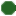Theorema

Summary

The Theorema project aims at extending current computer algebra systems by facilities for supporting mathematical proving. The present early-prototype version of the Theorema software system is implemented in Mathematica . The system consists of a general higher-order predicate logic prover and a collection of special provers that call each other depending on the particular proof situations. The individual provers imitate the proof style of human mathematicians and produce human-readable proofs in natural language presented in nested cells. The special provers are intimately connected with the functors that build up the various mathematical domains.
The long-term goal of the project is to produce a complete system which supports the mathematician in creating interactive textbooks, i.e. books containing, besides the ordinary passive text, active text representing algorithms in executable format, as well as proofs which can be studied at various levels of detail, and whose routine parts can be automatically generated. This system will provide a uniform (logic and software) framework in which a working mathematician, without leaving the system, can get computer-support while looping through all phases of the mathematical problem solving cycle.

Authors

Bruno Buchberger, Tudor Jebelean, Temur Kutsia, Wolfgang Windsteiger, Theorema group at RISC institute at JKU Linz, Austria

Statusofficially approved by the authors